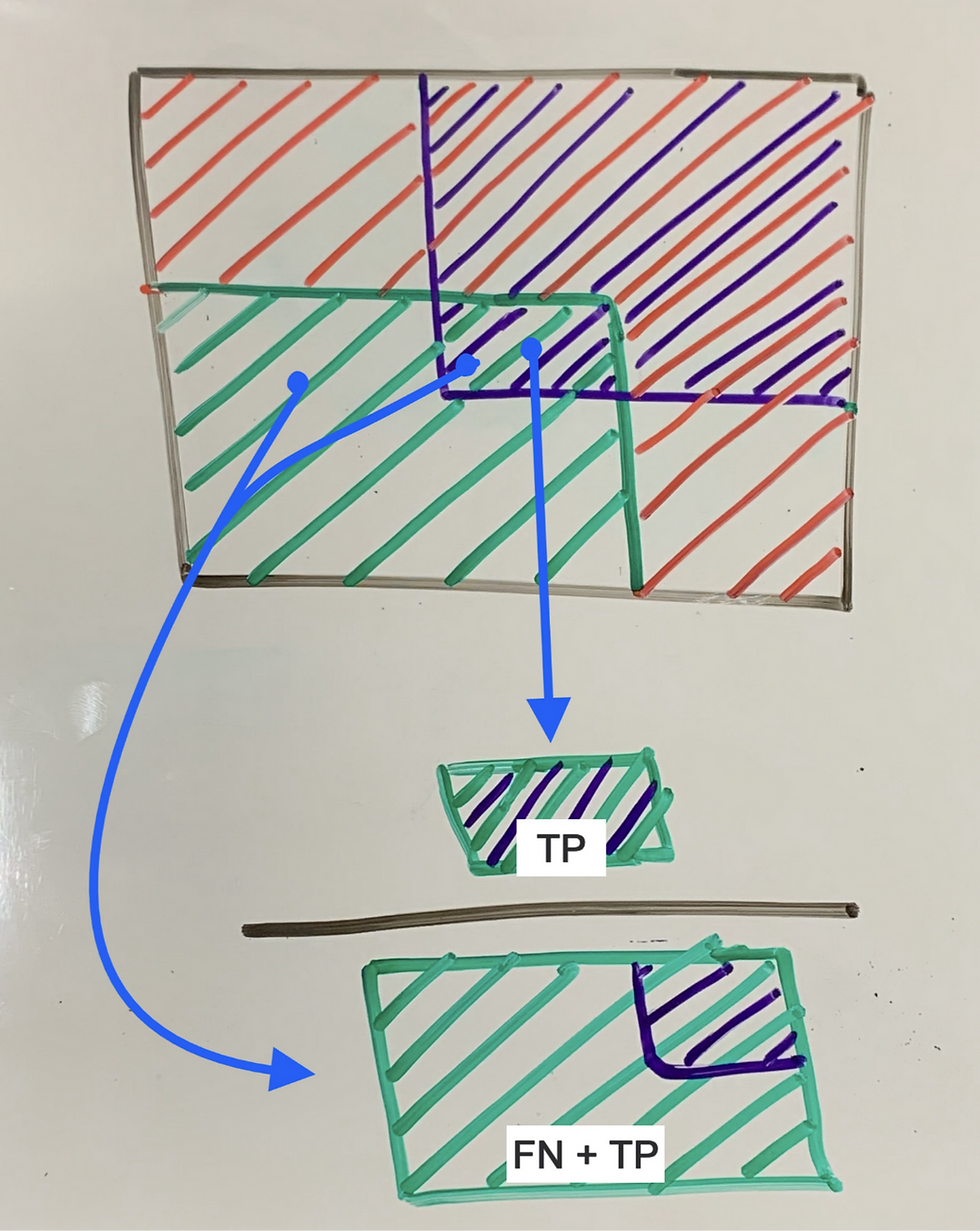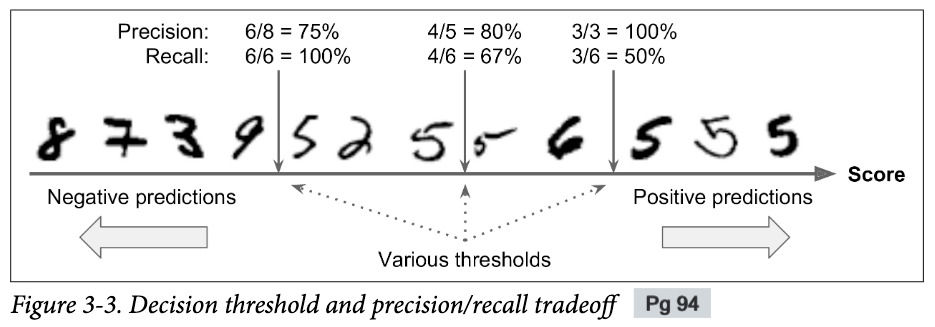top of page
Search
• Divakar V

# H.O.M.L Chapter-3 | Classification

Updated: Apr 17, 2021

Turning our attention to Classification systems.

Contents:

1. Precision

2. Recall

3. F1 score

4. PR curve

5. Multiclass (multinomial) Classification

6. Multilabel classification

7. Multioutput classification

## Performance Measure for Classification

Evaluating a classifier is often trickier than evaluating a regressor. Accuracy is generally not a preferred measure for classification. Imagine a skewed dataset where one of the classes occurs 90% of the time in the dataset. In such a case, if you simply predict this particular class always, you would achieve a 90% accuracy without doing anything. However, such a classifier won't be of any practical use.A better way to evaluate a classification model is to look at the confusion matrix. The idea is to count how many times one class is predicted as another class.

Confusion matrix provides valuable information and also helps us to come up with metrics to evaluate performance, namely, Precision and RecallI prefer to visualize it using Sets, like shown in the image. Here, Green & Red colors highlight the Actually occurring Positive class & actually occurring Negative class respectively. The Blue color shows the Predicted-Positive class, in other words, what the model thinks as the positive class.

TP (True Positive) - Actually True && predicted True

FP (False Positive) - Actually False but predicted True

TN (True Negative) - Actually False && predicted False

FN (False Negative) - Actually True but predicted FalsePrecision

Precision

From all the predicted outputs, how many of them are actually True.

```Precision = TP / (All the positive predictions                       by the model)

= TP / (TP + FP)```Recall

Recall

From all the actual Positive cases, how many were retrieved by the model.

```Recall = TP / (All the actual positive cases)

= TP / (TP + FN)```

## F1 score - Combining Precision & Recall

Instead of choosing one over the other, it would have been convenient if could combine the 2 scores into a single metric. This is what F1 score tries to do:

`F1 score = Harmonic mean of Precision and Recall`Regular Mean - Treats all values equally

Harmonic Mean - Gives more weightage to Small values.

`This means, you will only get F1 score if both Precision & Recall are high.	`

### Problem with F1-score:

1. If favors classifiers having similar precision and recall. However, you don't always want this.

2. In some cases, you might be just worried about Precision and not care about recall (e.g. adult website blocker - it's okay if some normal sites are blocked too but it's NOT Okay if an adult site is not blocked)

3. In some cases, you might want a high recall even if precision is poor. For example, a classifier to detect shoplifting in surveillance images - all the shoplifting scenarios should get detected even though there might be some false alarms.

4. There is a trade-off! We cannot have a high precision with a high recall (next section)Consider a classifier for detecting handwritten digit '5' which returns the scores as shown in the image. For each instance, classifier compares the score with a threshold value and depending upon that classifies it as Positive or Negative class.

High threshold value:Instances having high scores will be classified as Positive class. In other words, classifier will consider those cases as Positive class where it is highly confident. As a result of this, most of the predictions will be True Positive leading to a high Precision. However, the classifier will miss out many True positive instances because of lower scores than the threshold, leading to a lower Recall.

Lower threshold value:

Setting a lower threshold will retrieve more True positives instances but at the same time, many False positives will also be captured. Therefore, having a lower threshold value will generally lead to a better Recall but a poor Precision.

If we plot precision vs recall for various values of threshold, we get the graph shown below. This clearly highlights the Precision vs Recall trade-off (the graph is taken from the book itself, pg 95).

Another thing to note here is that precision can go down on increasing the threshold (although in general it goes up). Hence, the precision curve is little bumpier on the higher threshold values. This can be understood if you look at Figure 3-3 above and try different values of threshold. In contrast, recall always decreases on increasing the threshold.Another way to select a good precision/recall trade-off is to directly plot a precision vs recall (PR) graph for different threshold values (Fig 3-5). Notice how Precision suddenly starts to drop around 0.85 Recall. One has to be careful while choosing a particular value for Precision or Recall because there is a trade-off between the two.If you are aiming to achieve a very high value of Precision (say 99%), then you should always ask "At what Recall value!?"

## ROC Curve

ROC stands for Receiver Operating Characteristics and is a common way for evaluating Binary classifiers.

Like precision vs recall curve, ROC is the plot of True Positive Rate (TPR) vs False Positive Rate (FPR)

TPR = Recall

FPR = Ratio of negative instances that are incorrectly classified as positive.

= 1 - TNR (ratio of negative instances correctly classified as negative)

TPR is also referred to as Sensitivity. Whereas,

TNR is referred to as Specificity

Hence, ROC is a plot of Sensitivity vs (1 - Specificity), Figure 3-7.Different points in a given ROC curve corresponds to different Threshold values.

The dotted black line above (joining bottom-left corner to top-right) corresponds to a random classifier - one which randomly assigns a class to the input.

So, intuitively, a good classifier should stay as away as possible from this black dotted-line.

For comparing different classifiers using ROC curves, measure the Area Under the Curve (AUC). The ROC curve of a perfect classifier will pass through the top-left corner (0,1) and will have AUC value of 1. On the other hand, AUC value of a random classifier would be 0.5 and the curve would look like the black dotted line above.

### How to choose between ROC curve and PR curve?

1. When positive class is rare -> Choose PR curve

2. When you care more about False Positives (over False Negatives) -> Choose PR curve

3. Otherwise, choose ROC curve.

## When classification is more that just Binary

### 1. Muticlass (Multinomial) Classification

Classifiers to distinguish between more than 2 classes.

E.g. - SGD classifier, Random Forests, Naive Bayes

However, some are strictly binary classifiers, e.g. - Logistic Regression, SVM

Strategies to perform multiclass classification using multiple binary classifiers:

a.) one-versus-the-rest (OvR)

b.) one-versus-one (OvO)

For creating a Multiclass classifier using multiple binary classifiers for MNIST dataset (digits 0-9), the above strategies would look like this:

OvR - Create 10 binary classifiers for each of the 10 digits. For instance, a classifier to detect digit-3 would contain images of 3 as the positive class and all other images as the negative class. Similarly, create classifiers for other digits.

OvO - Train binary classifiers for every pair of the classes i.e. classifier for 0 vs 1, 0 vs 2, 0 vs 3... 1 vs 2, 1 vs 3... and so on. The predicted class would be the one which wins most number of duels. Total no. of classifiers would be:

`   N x (N-1) /2  ... where N is the total number of classes.`

OvR - Train less number of classifiers. But might be a problem when huge data.

OvO - Each binary classifier is trained on a part of data. Issue - too many classifiers.

`SVM scales poorly with size of training data. Hence, prefers OvO. Faster to train many small classifiers with OvO than to train less number of classifiers with OvR approach.`

Error Analysis for multiclass classifier can be done by plotting a confusion matrix.Right Image: Divide the original confusion matrix (left) by the number of class samples (sum of rows) to highlight errors

### 2. Multilabel Classification

Classification system that outputs multiple binary tags.

E.g. Consider we have 3 classes - Apple, Orange & Banana. We want to find out which all fruits are present in the given input image. A multilabel classification system would output something like [1,0,1], denoting presence of Apple & Banana (1) but absence of Orange (0).

3. Multioutput Classification

It is a generalization of Multilabel classification system where the system can output a non-binary label for each class i.e. a class can have more than 2 values.

In such system, output may look something like this (considering 4 classes):

`	[12, 35, 0, 2]`

Here, class-1 has an output value of 12, class-2 has a value of 35, class-3 of 0 and finally class-4 has a value of 2.

Takeaways:

1. Selecting good metrics for classification tasks

2. Picking appropriate Precision/Recall value

3. Compare classifiers

4. In general building a good classification system for a variety of tasks.

See All
bottom of page